Next: Azimuth of Ecliptic Ascension Up: Spherical Astronomy Previous: Terrestrial Climes

# Ecliptic Ascensions

Consider the rising, or ascension, of celestial objects at the eastern horizon, as viewed from a particular observation site on the earth's surface. If the observation site lies on the terrestrial equator then all celestial objects appear to ascend at right angles to the horizon. This process is known as right ascension. On the other hand, if the observation site does not lie at the equator then celestial objects appear to ascend at an oblique angle to the horizon. This process is known as oblique ascension. For the case of right ascension, it is easily demonstrated that all celestial objects with the same celestial longitude ascend simultaneously. Indeed, celestial longitude is generally known as right ascension'' because, in the case of right ascension, the celestial longitude of an object (in hours) is simply the time elapsed between the ascension of the vernal equinox, and the ascension of the object in question.

Let us now consider the ascension of points on the ecliptic. Applying Eq. (30) to a point on the celestial equator (i.e.,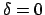) of right ascension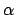, we obtain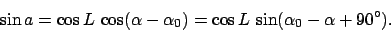(36)

It follows that we can write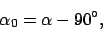(37)

whereis the right ascension of the point on the celestial equator which ascends at the eastern horizon (i.e.,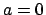and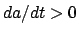) at the same time that celestial objects of right ascension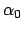are culminating at the meridian. Substituting this result into Eq. (32), we get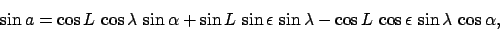(38)

which implies that ifandthen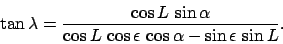(39)

This expression specifies the ecliptic longitude,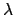, of the point on the ecliptic circle which ascends simultaneously with a point on the celestial equator of right ascension. Note, incidentally, that points on the celestial equator ascend at a uniform rate ofan hour at all viewing sites on the earth's surface (except the poles, where the celestial equator does not ascend at all). The same is not true of points on the ecliptic. Expression (39) can be inverted to give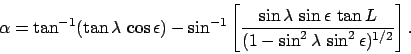(40)

The solution of Eq. (40) for observation sites lying above the arctic circle is complicated by the fact that, at such sites, a section of the ecliptic never sets, or descends, and a section never ascends. It is easily demonstrated that the section which never descends lies between ecliptic longitudes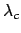and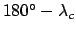, whereas the section which never ascends lies between longitudes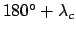and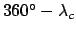. Here,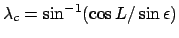. Points on the ecliptic of longitude,,, andascend simultaneously with points on the celestial equator of right ascension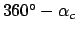,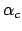,, and, respectively. Here,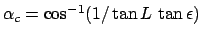.

Tables 5-17 list the ascensions of a series of equally spaced points on the ecliptic circle, as viewed from a set of observation sites in the earth's northern hemisphere with different terrestrial latitudes. The tables were calculated with the aid of formula (40). Let us now illustrate the use of these tables.

Consider a day on which the sun is at ecliptic longitude 14LE00 (i.e.,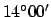into the sign of Leo). What is the length of the day (i.e., the period between sunrise and sunset) at an observation site on the earth's surface of latitude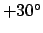? Consulting Table 8, we find that the sun ascends simultaneously with a point on the celestial equator of right ascension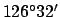. Now, the ecliptic is a great circle on the celestial sphere. Hence, exactly half of the ecliptic is visible from any observation site on the earth's surface. This implies that when a given point on the ecliptic circle is ascending, the point directly opposite it on the circle is descending, and vice versa. Let us term the directly opposite point the complimentary point. By definition, the difference in ecliptic longitude between a given point on the ecliptic circle and its complementary point is. Thus, the complimentary point to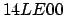is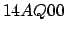. It follows thatascends at the same time thatdescends. In other words, the sun sets when 14AQ00 ascends. Consulting Table 8, we find that the sun sets at the same time that a point on the celestial equator of right ascension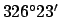rises. Thus, in the time interval between the rising and setting of the sun a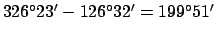section of the celestial equator ascends at the eastern horizon. However, points on the celestial equator ascend at the uniform rate ofan hour. Thus, the length, in hours, of the period between the rising and setting of the sun is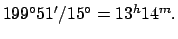In other words, the length of the day in question is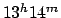.

The above calculation is slightly inaccurate for a number of reasons. Firstly, it neglects the fact that the sun is continuously moving on the ecliptic circle at the rate of about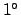a day. Secondly, it neglects the fact that the celestial equator ascends at the rate ofper sidereal, rather than solar, hour. A sidereal hour is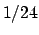th of a sidereal day, which is the time between successive upper transits of a fixed celestial object, such as a star. On the other hand, a solar hour isth of a solar day, which is the mean time between successive upper transits of the sun. A sidereal day is shorter than a solar day by 4 minutes. Fortunately, it turns out that these first two inaccuracies largely cancel one another out. Another source of inaccuracy is the fact that, due to refraction of light by the atmosphere, the sun is actuallybelow the horizon when it appears to rise or set. The final source of inaccuracy is the fact that the sun has a finite angular extent (of about half a degree), and that, strictly speaking, dawn and dusk commence when the sun's upper limb rises and sets, respectively. Of course, our calculation only deals with the rising and setting of the center of the sun. All in all, the above mentioned inaccuracies can make the true length of a day differ from that calculated from the ascension tables by up to 15 minutes.

Tables 5-17 also effectively list the descents of a series of equally spaced points on the ecliptic circle, as viewed from a set of observation sites in the earth's southern hemisphere with different terrestrial latitudes (which are minus those specified in the various tables). For instance, Table 6 gives the right ascensions of points on the celestial equator which set simultaneously with points on the ecliptic, as seen from an observation site at latitude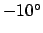.

Consider a day on which the sun is at ecliptic longitude 08SC00. Let us calculate the length of the day at an observation site on the earth's surface of latitude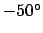? Consulting Table 10, we find that the sun sets simultaneously with a point on the celestial equator of right ascension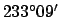. Now, the complementary point on the ecliptic to 08SC00 is 08TA00. Consulting Table 10 again, we find that this point sets simultaneously with a point on the celestial equator of right ascension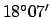. It follows that the sun rises simultaneously with the latter point. Thus, the time interval between the rising and setting of the sun is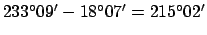time-degrees, or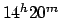.

The ascendent, or horoscope, is defined as the point on the ecliptic which is ascending at the eastern horizon. Suppose that we wish to find the ascendent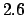hours after sunrise, as seen from an observation site of latitude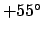, on a day on which the sun has ecliptic longitude 16SC00. Of course, knowledge of the ascendent at the time of birth is key to drawing up a natal chart in astrology. Hence, this type of calculation was of great importance to the ancients. Consulting Table 11, we find that, on the day in question, the sun rises simultaneously with a point on the celestial equator of right ascension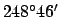. Now,hours corresponds to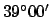. Thus, the ascendent rises simultaneously with a point on the celestial equator of right ascension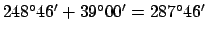. Consulting Table 11 again, we find that, to the nearest degree, the ascendent at the time in question has ecliptic longitude 13SG00.

Suppose, next, that we wish to find the right ascension,, of the point on the celestial equator which culminates simultaneously with a given point on the ecliptic of ecliptic longitude. From Eq. (33), we can see that if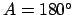then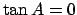, and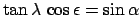, or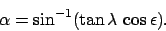(41)

However, this expression is identical to expression (40), when the latter is evaluated for the special case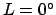. It follows that our problem can be solved by consulting Table 5, which is the ascension table for the case of right ascension. For instance, on a day on which the ecliptic longitude of the sun is 08TA00, we find from Table 5 that the right ascension of the point on the celestial equator which culminates simultaneously with the sun (i.e., which culminates at local noon) is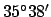. Moreover, this is the case for observation sites at all terrestrial latitudes. Note that we have effectively calculated the right ascension of the sun on the day in question.

Suppose, finally, that we wish to find the point on the ecliptic which culminates 7 hours after local noon on the aforementioned day. Since 7 hours corresponds to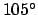, the right ascension of the point on the celestial equator which culminates simultaneously with the point is question is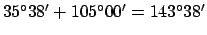. Consulting Table 5 again, we find that, to the nearest degree, the ecliptic longitude of the point in question is 21LE00.Next: Azimuth of Ecliptic Ascension Up: Spherical Astronomy Previous: Terrestrial Climes
Richard Fitzpatrick 2010-07-21